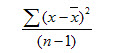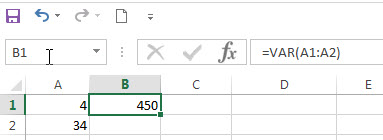# Excel VAR Function

This post will guide you how to use the VAR function with syntax and examples in Microsoft excel.

### Description

The Excel VAR function returns the variance of a population based on a sample of numbers. This function has been replaced by the VAR.S function in Excel 2010. The variance is used to identify the amount that the values vary from the average value across a set of values. And if you want to calculate the variance for an entire population, you can use the VARP function or the newer VAR.P function.

The VAR function is a build-in function in Microsoft Excel and it is categorized as a Statistical Function.

The VAR function is available in Excel 2016, Excel 2013, Excel 2010, Excel 2007, Excel 2003, Excel XP, Excel 2000, Excel 2011 for Mac.

### Syntax

The syntax of the VAR function is as below:

`=VAR(number1,[number2],...)`

Where the VAR function arguments are:

• number1-This is a required argument.  The first number or cell reference in a sample of a population.
• number2 -This is an optional argument.  Number arguments 2 to 255 corresponding to a sample of a population. And it can only accept up to 30 number arguments in Excel 2003 or older.

Note:

• The argument must be numbers or arrays or cell references that contain only numbers.
• The VAR function is only used for its arguments are a sample of the population. And if the argument represents the entire population, you need to use the VARP function to calculate the variance.
• If the argument contain the logical values and text representations of numbers and those values will be counted.
• Arguments that are error values or text that cannot be translated into numbers cause errors.
• VAR uses the following formula:### Excel VAR Function Examples

The below examples will show you how to use Excel VAR Function to calculate the variance for data that represents a sample of the population.

1# to get variance of a sample of numbers, using the following formula:

`=VAR (A1:A2)`### Related Functions

• Excel VARA Function
The Excel VARA function returns the variance of a population based on a sample of numbers, text, or logical values. The syntax of the VARA function is as below:= VARA (value1, [value2], ..)…
• Excel VARP Function
The Excel VARP function returns the variance of a population based on an entire population of numbers. The syntax of the VARP function is as below:=VARP(number1,[number2],…)…
• Excel VARPA Function
The Excel VARPA function returns the variance of a population based on an entire population of numbers, text, or logical values. The syntax of the VARPA function is as below:= VARPA (value1, [value2], ..)…